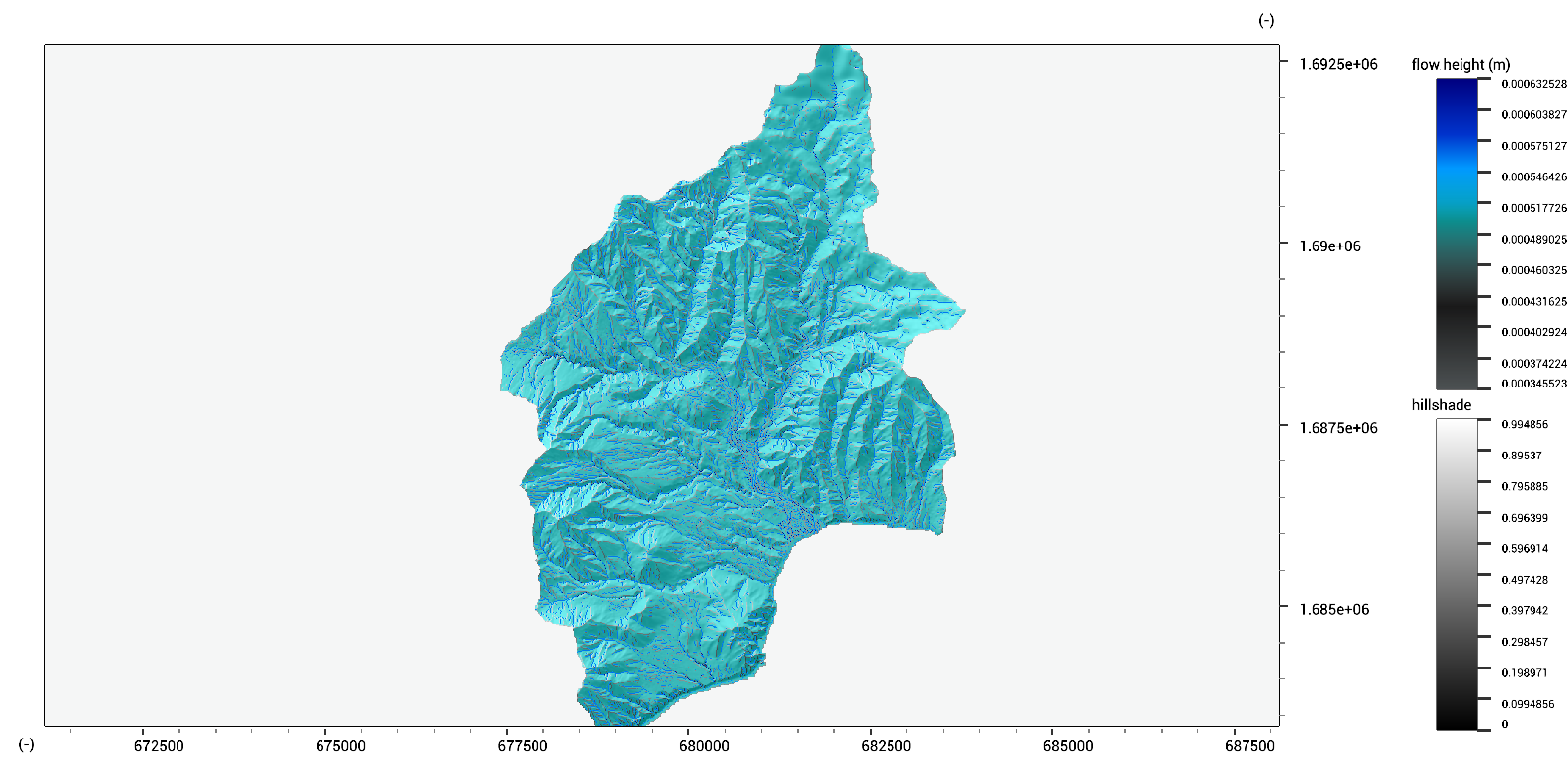LISEM Classic

LISEM

# Dynamic Models

With the functionality available in the scripting language, it is possible to write full, fast geo-spatial models. Several convenience functions have been provided for this. Additionally, some usefull physicall processes, such as water flow, have pre-made functions that do most of the work for you.

First, let us make a script where we load an elevation model.

void main()
{
Map dem = dem.tif;
}



Now, to simulat water flow, we have to make timesteps. We can add a loop that goes through the desired simulation period.

void main()
{
Map dem = dem.tif;

//we will simulate 1 hour, with timesteps of 6 seconds.
int n_steps = 60 *60/6; //nunmber of timesteps
float t = 0.0; //time in seconds
float dt = 6.0; //timestep in seconds

for(int i = 0; i < n_steps; i++)
{
t = t + 6;

//message the user if we are finished another minute
if( floor(t)%60 == 0)
{
Print("We are now at minute : " + ToString(t/60));
}
}
}


When you run this script, you should see a message for each minute that passes in the simulation. However, no work is being done yet. To simulate water flow, we will keep track of rainfall. We will simulate a rainfall intensity of 50 mm/hour for the first half hour.

void main()
{
Map dem = dem.tif;

//use the elevation model to create an empty map of identical size.
//this will be the water height, flow velocity in x and y direction
array<Map> flow = {dem * 0.0,dem * 0.0,dem * 0.0};

//we will simulate 1 hour, with timesteps of 6 seconds.
int n_steps = 60 *60/6; //nunmber of timesteps
float t = 0.0; //time in seconds
float dt = 6.0; //timestep in seconds
for(int i = 0; i < n_steps; i++)
{
t = t + dt;

if(t < 30*60)
{
//convert to meter/hour
flow += 50 * dt * 0.001 / (60*60);
}

//make a timestep of 6 second with the dynamic flow approximation
//the resutls overwrites the old data
flow = FlowDynamic(dem,dem*0.0 + 0.1,flow,flow,flow,6,0.2);

//message the user if we are finished another minute
if( floor(t)%60 == 0)
{
Print("We are now at minute : " + ToString(t/60));
}

//save the model results
h_final.tif = flow;

}

}


We can also visualize the result while the model is running. Before the time loop, we add a hillshade map as a background and the initial water height. Then, during the simulation, we keep updating the flow heights.

void main()
{
Map dem = dem.tif;

//use the elevation model to create an empty map of identical size.
//this will be the water height, flow velocity in x and y direction
array<Map> flow = {dem * 0.0,dem * 0.0,dem * 0.0};

UILayer hlay = AddViewLayer(flow,"flow height (m)");

//we will simulate 1 hour, with timesteps of 6 seconds.
int n_steps = 60 *60/6; //nunmber of timesteps
float t = 0.0; //time in seconds
float dt = 6.0; //timestep in seconds
for(int i = 0; i < n_steps; i++)
{
t = t + dt;

if(t < 30*60)
{
//convert to meter/hour
flow += 50 * dt * 0.001 / (60*60);
}

//make a timestep of 6 second with the dynamic flow approximation
//the resutls overwrites the old data
flow = FlowDynamic(dem,dem*0.0 + 0.1,flow,flow,flow,6,0.2);

//message the user if we are finished another minute
if( floor(t)%60 == 0)
{
Print("We are now at minute : " + ToString(t/60));
}

//update map viewer
ReplaceViewLayer(hlay,flow);

//save the model results
h_final.tif = flow;

}

}



The output to the map viewer can now be seen:Scripts like these can be easily extended to have specific boundary conditions (incoming waves) or feature additional processes (infiltration, either static or a model such as Green and Ampt).